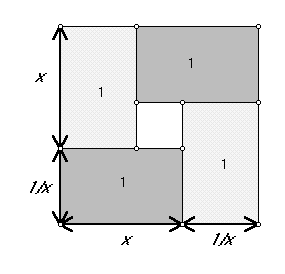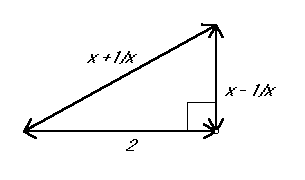Math 240                         Proof without Words #1
M. Flashman

The two figures below contain information that can be used to explain why the following inequality is true:

For each figure, give a written explanation based on the figure for the truth of the inequality.Figure 1Figure 2